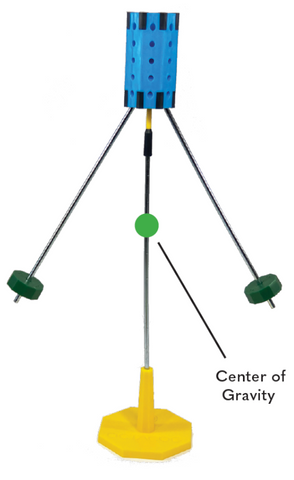# What does Center of Gravity Mean and How Does it Work with Octacog?

Sometimes, Octacog seems to defy gravity. But actually, Octacog is using gravity to balance in peculiar ways. By learning about center of gravity and equilibrium, you can start to predict how Octacog will balance. Center of gravity (used interchangeably with center of mass) is the point in an object or system where all mass is equally distant. Essentially, an average location of all the mass of an object.

In a symmetrical setup as shown, the Octacog core, with a balance point in the center, balances on the balance cap easily. It remains stable. The weights, at the end of the balance rods, are much lower than the balance point.The center of gravity or center of mass does not necessarily exist inside the object. In many cases, the center of gravity for Octacog is located in the air beneath Octacog. If you were to imagine where the center of gravity is in this setup, it would be in empty space, directly below the balance point, but above the level of the weights.

If the left weight is moved up the rod halfway, the weight is moving up and also to the right along the rod. At the same time, the center of gravity shifts up and to the right.  Because of this shift, Octacog will pivot on the balance point until the center of gravity is directly below the balance point and balance cap. At this point, Octacog reaches a balanced equilibrium. If another rod or weight is added, the center of gravity shifts toward that added part, disrupting the equilibrium and Octacog will pivot back toward equilibrium.

If the center of gravity rises too high or shifts too far to one side, Octacog will pivot too far and it will no longer balance. As you experiment with Octacog, you'll learn how changes in mass locations will change the center of gravity.